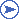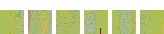### “Handling Floating-Point Exceptions in Numeric Programs”

Article by John R. Hauser, published in ACM Transactions on Programming Languages and Systems 18:2 (March 1996), pp. 139–174.
36 pages.

Abstract: There are a number of schemes for handling arithmetic exceptions that can be used to improve the speed (or alternatively the reliability) of numeric code. Overflow and underflow are the most troublesome exceptions, and depending on the context in which the exception can occur, they may be addressed either: (1) through a “brute force” reevaluation with extended range, (2) by reevaluating using a technique known as scaling, (3) by substituting an infinity or zero, or (4) in the case of underflow, with gradual underflow. In the first two of these cases, the offending computation is simply reevaluated using a safer but slower method. The latter two cases are cheaper, more automated schemes that ideally are built in as options within the computer system. Other arithmetic exceptions can be handled with similar methods. These and some other techniques are examined with an eye toward determining the support programming languages and computer systems ought to provide for floating-point exception handling. It is argued that the cheapest short-term solution would be to give full support to most of the required (as opposed to recommended) special features of the IEC/IEEE Standard for Binary Floating-Point Arithmetic. An essential part of this support would include standardized access from high-level languages to the exception flags defined by the standard. Some possibilities outside the IEEE Standard are also considered, and a few thoughts on possible better-structured support within programming languages are discussed.Adobe PDF document, `1996_Hauser_FloatingPointExceptions.pdf`.

### Notes

• Section 3.3, page 151: At the bottom of the page, the line

for all x and all zΩ.
should be
for all x, z with z = 9x2Ω.

• Section 3.4, page 157: The following sentence is not completely correct:

In the “fast” mode, zero is substituted on underflow, and subnormal inputs are identified with zero.
Contrary to my expectations, Digital’s Alpha system outright refuses to accept subnormal inputs in “fast” mode. The program is aborted.

(This policy has created trouble in at least one case when someone at U.C. Berkeley was attempting to use an Alpha to process floating-point data created on another machine.)

### Selected responses to reviewer comments

As usually happens, some interesting concerns were voiced during the review of my paper for publication. I have listed below a few selected reviewer comments, followed by the responses I gave at the time. The comments chosen are ones that I disputed and thus were never reflected in the final paper. All the comments I’ve listed actually came from a single particularly astute reviewer. Be aware that the fact that the paper was eventually published does not imply that the reviewer was impressed by my responses (assuming he even saw them).

• Section 4, page 158: This is not the mathematical definition of a pole. The mathematical definition requires that f(x) be defined in a whole punctured disk, ruling out log(x). It also requires that the singularity be like 1/polynomial, ruling out things like exp(1/x) at x = 0.

The usual mathematical definition (“whole punctured disk,” etc.) is for the complex domain. Since I only ever talk about the real domain and real functions, I want the equivalent concept for the reals, for which a term does not already exist as far as I know. At least one professor in the U.C. Berkeley Mathematics Department (W. Kahan) calls my use of the term pole for the reals “perfectly reasonable.” I am willing to accept another term if the reviewer can suggest one. No one I spoke with thought any other term would be better.

I have modified the wording to imply that I am defining the term pole for real functions. To avoid unhelpful detail, my definition is not stated with mathematical precision; nevertheless, whether the real function exp(1/x) would be said to have a pole at x = 0 doesn’t affect the discussion in the paper since the function is necessarily undefined (indeterminate) at 0.

• Section 4, page 160: I didn’t find the discussion of signed zero very balanced. The author mentions that adding signed zero preserves the identity 1/(1/x) = x. But it also destroys identities, such as x = y if and only if 1/x = 1/y.

I’m not sure how to address this concern. I feel I do try to show problems with signed zeros, including pointing out a useful theorem that is true for unsigned zero/infinity and not true for signed zeros/infinities.

In that part of the paper that discusses signed zeros, the existence of signed infinities is being hypothesized. Given that, there are two options: Make 1/0, 1/+inf, and 1/−inf all be undefined, or have signed zeros. The first option is more elegant; the second option is more practical for doing computation. Traditional real analysis chooses the first road; the committee that wrote the IEEE Standard took the other. I discuss the more practical road, firstly because it is more practical, and secondly because it has been enshrined by the IEEE Standard.

(Note that when 1/+inf = 1/−inf = 0 (unsigned), the proposition “x = y if and only if 1/x = 1/y” is violated for x = +inf and y = −inf. Hence, 1/+inf and 1/−inf must be undefined if this identity is to be preserved.)

• Section 5, page 161: I find the assertion that the function sin(x)/x has a singularity at x = 0 to be hairsplitting. I’d wager \$5 that 90% of mathematicians would claim that sin(x)/x does not have a singularity at x = 0, since to them it would be the name of the function defined as: f(0) = 1, and f(x) = sin(x)/x elsewhere.

In computer programs, functions are effectively defined by the expressions that evaluate them. If a `sinc` subroutine is coded as ‘`sin(x)/x`’, the subroutine will fail for x = 0. Mathematicians may automatically eliminate removable singularities in expressions like sin(x)/x, but computers won’t.

I have replaced the word function by expression in an attempt to be more precise.

• Section 5, page 162: Concerning the discussion of 00, the Knuth argument (which is probably more accessible in his book Concrete Mathematics than the given reference to the American Mathematical Monthly) does not seem completely relevant to me. It applies to the function xn when n is an integer. That reasoning does not necessarily carry over to the function xy where y is a real number.

To quote from Concrete Mathematics (Graham, Knuth, and Patashnik):

Some textbooks leave the quantity 00 undefined, because the functions x0 and 0x have different limiting values when x decreases to 0. But this is a mistake. We must define
x0 = 1, for all x,
if the binomial theorem is to be valid when x = 0, y = 0, and/or x = −y. The theorem is too important to be arbitrarily restricted! By contrast, the function 0x is quite unimportant. (See  for further discussion.)
Reference 220 is Knuth’s American Mathematical Monthly article that I cite.

I note that Graham et al. don’t mention any distinction between the integers and reals in their argument. (Nor does Knuth in his article.) The comment about x0 and 0x having different limiting values as x approaches 0 would be meaningless if the x in 0x was assumed to be an integer. Thus I infer that Knuth believes 00 ought to be 1 even if the exponent is taken from among the reals.

I have to wonder what value the reviewer would assign to 00 assuming:

1. the exponent is a rational number.
2. the exponent comes from the set of (unbounded) floating-point numbers:
{ a×2b : a, b integers, |a| < 2N } for some integer N.
3. the exponent comes from the set of (unbounded) fixed-point numbers:
{ a×2E : a an integer } for some integer E.

Where is the dividing line between 00 being undefined and 00 = 1? Notice that the set of unbounded fixed-point numbers is exactly the set of integers if E = 0. (Notice also that the floating-point numbers are a subset of the rationals.)

It is mathematically acceptable to have 00 be 1 when “the exponent is an integer” and undefined when “the exponent is a real number”. For doing computation, however, I believe it is more useful to adopt as much as possible a model with exactly one set of numbers and one definition for any operation on those numbers. In this model, every integer is also at the same time a rational number, and every rational number is also at the same time a real. The identity of the number 0 is thus independent of what sets it appears in (N, Z, Q, R). Likewise, arithmetic operations are not dependent on the sets (domains) from which their operands are selected, be they Z, Q, R, or whatever. It then becomes meaningless to talk about the value of 00 being different depending on whether the exponent is integer or real. The exponent 0 is always both integer and real. Therefore, if 00 must be 1 for the integers, it is necessarily 1 for the reals.

Unfortunately, it would be inappropriate for me to lengthen the discussion in the paper. I would cite better references if only I knew of them.John Hauser, 2020 March 8